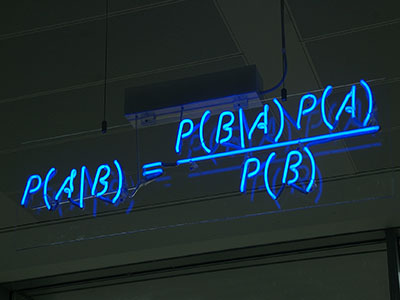ΓραμματείαSecretariat: 2410 684574 | ΦοιτητικάStudents: 2410 684387 g-ds@uth.gr
Select Page

# Probability and Statistics

Υ202

2

#### Hours/Week - ECTS

5 – 6### Indicative Module Content

• Basic probability concepts and definitions
• Combinatorial analysis
• Random variables (discrete and continuous)
• Theoretical Probability distributions
• Binomial, Normal, Poisson, Bernoulli, Gamma, Exponential
• Basic concepts of Statistics
• Estimation theory
• Space of reliance
• Random variable functions.